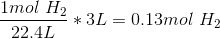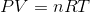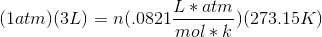# AP Chemistry : Properties of Gases

## Example Questions

← Previous 1

### Example Question #1 : Properties Of Gases

In reality, the pressure of a gas is slightly less than the pressure predicted for an ideal gas. This is because __________.

repulsive interactions between gas molecules cause harder collisions with the vessel wall

the ideal pressure takes the volume of the molecules into consideration

intermolecular attractions slow the gas molecules before colliding with the vessel wall

the ideal pressure takes attractive intermolecular forces into consideration

intermolecular attractions slow the gas molecules before colliding with the vessel wall

Explanation:

Ideal gas pressure only takes the repulsive forces between gas molecules into consideration. The truth is, gas molecules can also exhibit attractive forces with one another (London dispersion forces). This attractive force pulls the molecules inward and slows their velocity before striking the wall of the container. As a result, real gases exert slightly less pressure compared to the ideal pressure.

### Example Question #1 : Properties Of Gases

A gas is behaving ideally

2 mols of the gas would have what volume at STP?

0 L
11.2 L
22.4 L
44.8 L
Explanation:

use PV = nRT

V = nRT / P  ; must covert T into K

V = (2)(0.0821)(273)/ 1

= 44.8 L

### Example Question #1 : Properties Of Gases

Which of the following situations would most likely cause gases to deviate from ideal behavior?

High pressure and low temperature

Low pressure and low temperature

Gases never deviate from ideal behavior

High pressure and high temperature

Low pressure and high temperature

High pressure and low temperature

Explanation:

At high pressure and low temperature two things are happening that will cause gases to deviate from ideal behavior.  At low temperature the individual gas molecules are moving slower. As the pressure is increased the individual molecules are being pushed closer to one another.  Thus, when the gas molecules are closer together and moving at reduced speeds they are more likely to interact with one another.  Ideal gas behavior is dependent upon an absence of intermolecular interaction between the gas molecules.  Therefore, the increased likelihood of intermolecular interactions between the gas molecules at increased pressure and decreased temperature is likely to cause gases to deviate from ideal behavior.

### Example Question #44 : Gases

Two balloons are filled with gas at STP.  One is filled with hydrogen gas, the other with neon gas.  The Volume of the balloon filled with hydrogen gas is 22.4 L, the balloon filled with neon is 44.8 L.

There are more atoms in which balloon?

The Baloon filled with hydrogen gas

Both balloons contain the same number of atoms

The balloon filled with neon gas

Both balloons contain the same number of atoms

Explanation:

Hydrogen gas is a diatomic gas, so the molecules that are filling the balloon exist as H2.  Neon since it is a noble gas exists as a monoatomic gas, so the molecules that are filling the neon balloon exist as Ne.  The volumes given allow us to calculate the amount of each gas in moles.  At STP one mole of gas occupies 22.4 L, so there is one mole of hydrogen gas and there are two moles of neon gas.  One mole of hydrogen gas indicates that there are actually two moles of hydrogen atoms in the balloon.  Two moles of neon gas indicates that there are two moles of neon gas present in the balloon because neon exists as a monoatomic gas.  Thus there are two moles of atoms in each balloon.

### Example Question #1 : Properties Of Gases

Which of the following is a characterization of an ideal gas?

I. Low concentration

II. High Pressure

III. Elastic Collisions

II and III only

I only

I and III only

I, II, and III

I and II only

I and III only

Explanation:

An ideal gas is most likely low concentration of identical molecules and low pressure. The molecules move randomly and collisions are completely elastic.

### Example Question #46 : Gases

A 3 liter container contains hydrogen gas. Assuming standard temperature and pressure (STP) and ideal conditions, how many moles of hydrogen gas are present in the container?

6mol

0.17mol

0.13mol

0.33mol

0.13mol

Explanation:

At STP, one mole of an ideal gas occupies 22.4L. You should know this value for the exam.Another approach would use the ideal gas law:. Since we know that the container is at STP, we know that the container has a temperature of 273.15K and has a pressure of 1atm.

Adding the other known variables, the equation becomesSolving for n, we find that there is 0.13 moles of hydrogen gas in the container.

### Example Question #1 : Properties Of Gases

In reality, the volume of a gas is slightly larger than the ideal volume. This is because __________.

ideal volume does not incorporate pressure's effect on the size of the container

ideal volume does not take the volume of the gas molecules into consideration

ideal volume does not take the width of the container into consideration

ideal volume already uses the volume of the gas molecules

ideal volume does not take the volume of the gas molecules into consideration

Explanation:

One of the key charactersitics of an ideal gas is that gas molecules have no volume. This is obviously not the case, and the volume of the molecules must be added to the ideal volume. As a result, the real volume is slightly larger than the ideal volume.

### Example Question #48 : Gases

Which of the following conditions would cause a gas to act the most like an ideal gas?

Low pressure and high temperature

High pressure and high temperature

Low pressure and low temperature

High pressure and high temperature

Low volume and low temperature

Low pressure and high temperature

Explanation:

An ideal gas acts as if there are no interactions between the gaseous molecules during their rapid movements. At high temperature and low pressure the particles of the gas will not interact very much, as they will have high energy and will move around very fast. The faster the movement, the less time and contact the particles have with one another. The slower the particles are moving, the more they are starting to act like a liquid, and less like an ideal gas. High pressure will condense the particles, while low pressure will allow them to move freely. High temperature will add energy to speed the particles, while low temperature will slow them down.

### Example Question #1 : Properties Of Gases

You place some balloons in your car, and leave them to sit as the car is heated by the sun. What effect would this have on the gases inside the helium balloons?

Assume the balloons are fully elastic.

The volume of the balloons will increase

The pressure inside the balloons will increase

The volume of the balloons will decrease

The temperature of the gases surrounding the balloon will decrease

There will be more molecules of helium inside the balloons

The volume of the balloons will increase

Explanation:

We can predict the result of the heating by using the ideal gas law:We know that, which is a constant, will never change. We also know that, the number of moles of helium inside the balloons, will stay the same since no gas is added or removed from the sealed balloons.

The variables in this case are pressure, volume, and temperature. We know that the temperature will increase because the sun is heating up the car. This leaves us with pressure and volume. Pressure will not increase because the balloons are elastic; as the gas expands, the balloons expand as well without increasing the pressure. In this scenario, only the volume will increase.

### Example Question #50 : Gases

Which of the following conditions would result in the deviation of a gas from ideality?

A gas would behave ideally regardless of temperature and pressure

Low temperature and low pressure

High temperature and low pressure

High temperature and high pressure

Low temperature and high pressure

Low temperature and high pressure

Explanation:

Under high pressure, the volume of the gas molecules would no longer be negligible—one of the assumptions for an ideal gas. In addition, at low temperature, the molecules would have less kinetic energy, and intermolecular forces could take affect—another assumption of an ideal gas is that there are no intermolecular attractions or repulsions.

At low pressure and high temperature, gases behave more like gases and liquids are more likely to boil (convert to gas). At high pressure and low temperature, gases behave less ideally and can condense (convert to liquid).

← Previous 1

### All AP Chemistry Resources